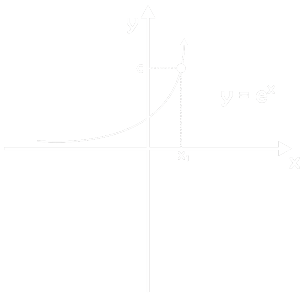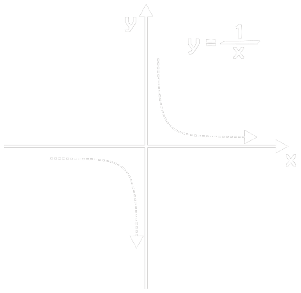Home > Math Shortcuts > Equations Formula

# Equations Formula

## Equations Formula:

Here in this page we will discuss all basic concept of Equations Formula. So, let’s get started.

What is linear equation ?
when equation between two variables that gives a straight line when plotted on a graph its called linear equation.ax + b = 0,   a ≠ 0 ⇒    x = – b / a

## Two Linear Equations :

#### a1 x + b1 y = c1                 a1 / a2 ≠ b1 / b2 a2 x + b2 y = c2##• ax = c,        a > 0
• If c > 0, a ≠ 1 :  So one root x = loga c
If c = 1, a = 1, So many roots,
If c ≠ 1, a = 1, So no solution,
If c ≤ = 0, no So no solution,

## Logarithmic Equations :logaa x = c (a > 0, a ≠ 1)
x = a c

## g(x) = f(x – c)## h(x) = -f(x)### Exponential Inequation :

• αA(x) > aB(x)
• α > 0, A(x) > B(x)
• 0< α < 1, A(x) < B(x)

### Logarithmic Inequation :

loga α(x) = loga β(x)

• a > 1:
• β(x) > 0 , α(x) > β(x)

• 0<a<1:
• α(x) > 0, α(x) < β(x)

## Square Root :## Reciprocal :### Cubic :## Sec :## Cosec :## Absolute :## Constant :1.